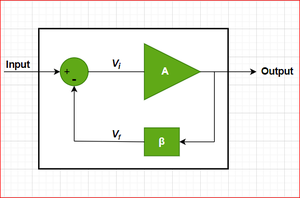# Need of Negative Feedback in Amplifiers

• Difficulty Level : Medium
• Last Updated : 05 Feb, 2021

An Amplifier is simply an electronic device that is used to increase the strength of the input signal. In this process of amplification, even the disturbances or noise present in the input signal also get amplified.

That’s the reason why we use negative feedback in amplifiers, a portion of the output signal in a phase opposite to the input signal is fed to the input of the amplifier which significantly reduces the noise level in the output signal.Block diagram of an amplifier with negative feedback

Negative feedback in amplifiers has the following advantages:

1. Stabilizes Amplifier Gain

The negative feedback stabilizes the gain of the amplifier by reducing the dependence of amplifier gain on various transistor parameters or variation in the supply voltage.

Gvf = Gv / (1 + β . Gv)

Here Gvf = resultant amplifier gain with negative feedback

Gv = amplifier gain without feedback

and β is the feedback fraction or feedback ratio

The above equation clearly shows that the resultant amplifier gain with negative feedback depends mainly on feedback fraction or feedback ratio.

2. Reduces Non-linear Distortion

The use of negative feedback also reduces the non-linear distortion level in the large signal amplifiers.

Dvf = D / (1 + β . Gv)

Here Dvf = resultant distortion level with negative feedback

D = distortion level without feedback

Gv = amplifier gain without feedback

and β is the feedback fraction or feedback ratio

The above equation clearly shows that the distortion level in the case of an amplifier with negative feedback is reduced by a factor of (1 + β . Gv).

3. Increases Circuit Stability

The output of an amplifier without negative feedback is affected by the variations in the temperature, frequency, or amplitude of the signal which further changes the gain of the amplifier and as result, we get a distorted signal in the output. Hence, the negative feedback is applied so that the gain of the amplifier is stabilized.

4. Increases Input Impedance/Resistance

The use of negative feedback increases the input impedance or resistance of the amplifier.

Z’in = Zin . (1 + β . Gv)

Here Z’ = resultant input impedance with negative feedback

Z = input impedance without feedback

Gv = amplifier gain without feedback

and β is the feedback fraction or feedback ratio

The above relation shows that the input impedance of the amplifier with negative feedback is increased by the factor of (1 + β . Gv).

5. Decreases Output Impedance/Resistance

The use of negative feedback decreases the output impedance or resistance of the amplifier.

Z’o = Zo / (1 + β . Gv)

Here Z’o = resultant output impedance with negative feedback

Zo = output impedance without feedback

Gv = amplifier gain without feedback

and β is the feedback fraction or feedback ratio

The above relation shows that the output impedance of the amplifier with negative feedback is decreased by the factor of (1 + β . Gv).

6. Reduces Noise Level

The negative feedback which we apply to the amplifiers is in the opposite phase to that of the applied input signal, hence it cancels out the noises which are introduced in the output signal by the amplifier circuit. As a result, we get the output signal with a reduced noise level.

7. Improves Frequency Response & Bandwidth

The negative feedback which we apply to the amplifiers is a resistive network hence the gain of the amplifier with negative feedback is independent of signal frequency. As a result, the gain becomes constant over a wide range of signal frequencies in this way the frequency response of the amplifier with negative feedback is improved.

f’cf = fcf . (1 + β . Gv)

Here f’cf = resultant cut-off frequency with negative feedback

fcf = cut-off frequency without feedback

Gv = amplifier gain without feedback

and β is the feedback fraction or feedback ratio

The above relation clearly shows that the resultant cut-off frequency of the amplifier with negative feedback is increased by the factor of (1 + β . Gv). Hence, we get a more usable bandwidth in the case of an amplifier with negative feedback.

8. More Linear Operations

In the case of an ordinary amplifier, we get a very high value of the output signal even for a small value of the input signal hence the output signal is not proportional to the applied input signal. But in the case of an amplifier with negative feedback, every parameter is controlled by the applied feedback network. Hence in the case of an amplifier with negative feedback, the relation between the output and the input signal is more linear.

As we have discussed the various advantages of using negative feedback in amplifiers, it is important to know the main disadvantage of using negative feedback in amplifies, that is the overall gain of the amplifier is reduced by the factor of (1 + β . Gv).

My Personal Notes arrow_drop_up